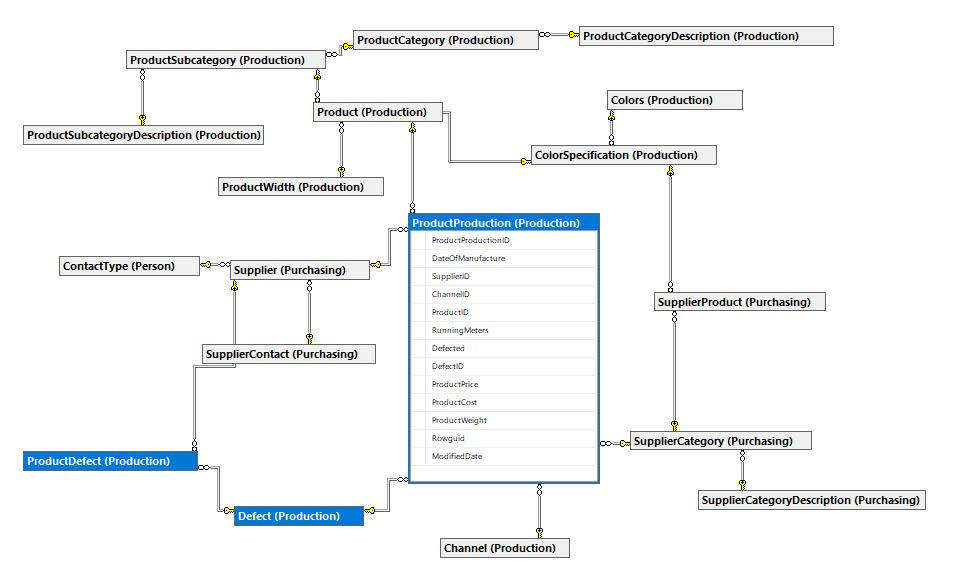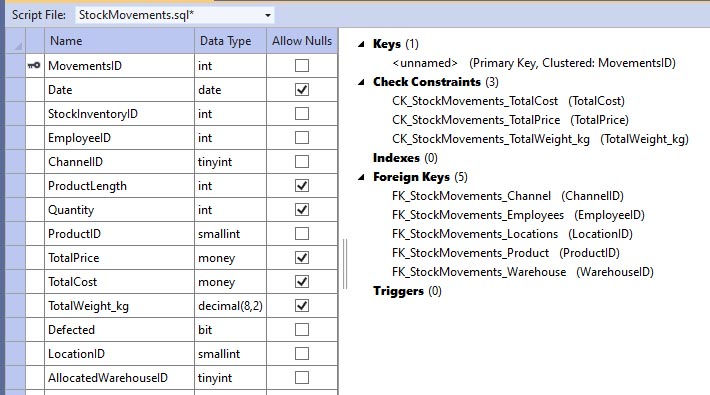top of page
Search
• Marek Vavrovic

# Huffner Textile - Relational database

HuffnerTextileRD Database Project
.zip

Database Design Objective

• Eliminate Data Redundancy: the same piece of data shall not be stored in more than one place. This is because duplicate data not only waste storage spaces but also easily lead to inconsistencies.

• Ensure Data Integrity and Accuracy: is the maintenance of, and the assurance of the accuracy and consistency of, data over its entire life cycle, and is a critical aspect to the design, implementation, and usage of any system which stores, processes, or retrieves data.

There are four stages of an RDM

• Relations and attributes − The various tables and attributes related to each table are identified. The tables represent entities, and the attributes represent the properties of the respective entities.

• Primary keys − The attribute or set of attributes that help in uniquely identifying a record is identified and assigned as the primary key.

• Relationships −The relationships between the various tables are established with the help of foreign keys. Foreign keys are attributes occurring in a table that are primary keys of another table. The types of relationships that can exist between the relations (tables) are One to one, One to many, and Many to many

• Normalization − This is the process of optimizing the database structure. Normalization simplifies the database design to avoid redundancy and confusion. The different normal forms are as follows:

• First Normal Form (1NF)

• Second Normal Form (2NF)

• Third Normal Form (3NF)

• Boyce-Codd Normal Form or Fourth Normal Form (BCNF of 4NF)

• Fifth Normal Form (5NF)

• Sixth Normal Form (6NF)

E-R Modelling Process

• Identify the entities that your database must represent

• Determine the cardinality relationships among the entities and classify them as one of

• One-to-one (e.g., a parcel has one address)

• One-to-many (e.g., a parcel may be involved in many fires)

• Many-to-many (e.g., parcel sales: a parcel may be sold many times by different owners, and an individual owner may sell many parcels)

• Draw the entity-relationship diagram

• Determine the attributes of each entity

• Define the (unique) primary key of each entity

• Define the relationships between primary keys in one table and foreign keys in another

Database description: ER Diagrams

Schemas: Production, Warehouse, Purchasing, Sales, Person ,HumanResources.

Database consists of seven transactional tables:

Table is recording Supplier transactions.[Production].[ProductProduction] and [Production].[ProductDefect]

Production.Defect is a bridge table. Bridge tables are used to resolve many-to-many relationships between two tables. Table is storing data from production.[Warehouse].[StockInventory]

This transactional table contains data about products coming out from production.[Sales].[StockMovements]

Table contains information about historical product movements. The transactions are connected to the Production, Warehouse and Sales processes.[Sales].[SubDepotSales]

Table contains information about sub depot sales.[Sales].[ClientSales]

Data in this table are related to the client sales.Transactional tables definition

(Primary keys, Foreign keys, Relationships, Constrains)

Table designCheck Constraints

CONSTRAINT [CK_SupplierOrderDetail_FreightAmount] CHECK ([FreightAmount]>=(0.00)),

CONSTRAINT [CK_SupplierOrderDetail_Quantity] CHECK ([Quantity]>=(0)),

CONSTRAINT [CK_SupplierOrderDetail_RollSize] CHECK ([RollSize]>=(0)),

CONSTRAINT [CK_SupplierOrderDetail_SubTotal] CHECK ([SubTotal]>=(0.00)),

CONSTRAINT [CK_SupplierOrderDetail_Tax] CHECK ([SaleTax]>=(0.00)),

CONSTRAINT [CK_SupplierOrderDetail_TaxAmount] CHECK ([TaxAmount]>=(0.00)),

CONSTRAINT [CK_SupplierOrderDetail_TotalCost] CHECK ([TotalCost]>=(0.00)),

CONSTRAINT [CK_SupplierOrderDetail_TotalDue] CHECK ([TotalDue]>=(0.00)),

CONSTRAINT [CK_SupplierOrderDetail_TotalWeight_kg] CHECK ([TotalWeight_kg]>=(0.00)),

CONSTRAINT [CK_SupplierOrderDetail_UnitCost] CHECK ([UnitCost]>=(0.00)),

CONSTRAINT [CK_SupplierOrderDetail_UnitPrice] CHECK ([UnitPrice]>=(0.00)),

[Production].[ProductProduction]

Table designCheck Constraints

CONSTRAINT [CK_Product_Defected] CHECK ([Defected]>=(0.00)),

CONSTRAINT [CK_ProductProduction_ProductCost] CHECK ([ProductCost]>=(0.00)),

CONSTRAINT [CK_ProductProduction_ProductPrice] CHECK ([ProductPrice]>=(0.00)),

CONSTRAINT [CK_ProductProduction_ProductWeight] CHECK ([ProductWeight]>=(0.00))

[Production].[ProductDefect]

Table designCheck Constraints

CONSTRAINT [CK_ProductDefect_PercentageOfDefects] CHECK ([PercentageOfDefects]>=(0.00))

[Warehouse].[StockInventory]

Table designCheck Constraints

CONSTRAINT [CK_StockInventory_Defected] CHECK ([Defected]>=(0) AND [Defected]<=(1)),

CONSTRAINT [CK_StockInventory_ProductLength] CHECK ([ProductLength]>=(0)),

CONSTRAINT [CK_StockInventory_Quantity] CHECK ([Quantity]>=(0)),

CONSTRAINT [CK_StockInventory_TotalCost] CHECK ([TotalCost]>=(0.00)),

CONSTRAINT [CK_StockInventory_TotalPrice] CHECK ([TotalPrice]>=(0.00))

[Sales].[StockMovements]

Table designCheck Constraints

CONSTRAINT [CK_StockMovements_TotalCost] CHECK ([TotalCost]>=(0.00)),

CONSTRAINT [CK_StockMovements_TotalPrice] CHECK ([TotalPrice]>=(0.00)),

CONSTRAINT [CK_StockMovements_TotalWeight_kg] CHECK ([TotalWeight_kg]>=(0.00))

[Sales].[SubDepotSales]

Table designCheck Constraints

CONSTRAINT [CK_SubDepotSales_Defected] CHECK ([Defected]>=(0) AND [Defected]<=(1)),

CONSTRAINT [CK_SubDepotSales_Discount] CHECK ([Discount]>=(0.00) AND [Discount]<=(1.00)),

CONSTRAINT [CK_SubDepotSales_ProductLength] CHECK ([ProductLength]>=(0)),

CONSTRAINT [CK_SubDepotSales_Quantity] CHECK ([Quantity]>=(0)),

CONSTRAINT [CK_SubDepotSales_ShipDate] CHECK ([ShipDate]>=[OrderDate] AND [ShipDate]>=[DateOfManufacture]),

CONSTRAINT [CK_SubDepotSales_SubTotal] CHECK ([SubTotal]>=(0.00)),

CONSTRAINT [CK_SubDepotSales_TaxAmount] CHECK ([TaxAmount]>=(0.00)),

CONSTRAINT [CK_SubDepotSales_TotalDue] CHECK ([TotalDue]>=(0.00))

[Sales].[ClientSales]

Table designCheck Constraints

CONSTRAINT [CK_ClientSales_Defected] CHECK ([Defected]>=(0) AND [Defected]<=(1)),

CONSTRAINT [CK_ClientSales_DeliveryDate] CHECK ([DeliveryDate]>=[ShipDate] AND [DeliveryDate]>=[DateOfManufacture]),

CONSTRAINT [CK_ClientSales_ProductLength] CHECK ([ProductLength]>=(0)),

CONSTRAINT [CK_ClientSales_SubTotal] CHECK ([SubTotal]>=(0.00)),

CONSTRAINT [CK_ClientSales_TaxAmount] CHECK ([TaxAmount]>=(0.00)),

CONSTRAINT [CK_ClientSales_TotalDue] CHECK ([TotalDue]>=(0.00)),

CONSTRAINT [CK_ClientSales_UnitPrice] CHECK ([Quantity]>=(0))

Normalization

"Database normalization is the process of structuring a relational database in accordance with a series of so-called normal forms to reduce data redundancy and improve data integrity. It was first proposed by Edgar F. Codd as part of his relational model.

Normalization entails organizing the columns (attributes) and tables (relations) of a database to ensure that their dependencies are properly enforced by database integrity constraints. It is accomplished by applying some formal rules either by a process of synthesis (creating a new database design) or decomposition (improving an existing database design)."

The database normalization process can be divided into following types:

1. First Normal Form (1NF)

2. Second Normal Form (2NF)

3. Third Normal Form (3NF)

4. Boyce-Codd Normal Form or Fourth Normal Form (BCNF of 4NF)

5. Fifth Normal Form (5NF)

6. Sixth Normal Form (6NF)

### First Normal Form (1NF)

• Data is stored in tables with rows that can be uniquely identified by a Primary Key.

• Data within each table is stored in individual columns in its most reduced form.

• There are no repeating groups.

### Second Normal Form (2NF)

• All the rules from 1NF must be satisfied.

• Only those data that relates to a table’s primary key is stored in each table.

### Third Normal Form (3NF)

• All the rules from 2NF must be satisfied.

• There should be no intra-table dependencies between the columns in each table.